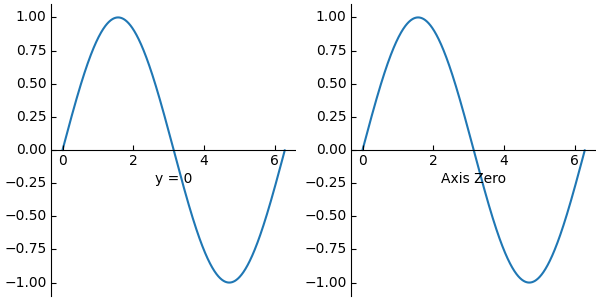# Custom spines with axisartist#

This example showcases the use of axisartist to draw spines at custom positions (here, at y = 0).

Note, however, that it is simpler to achieve this effect using standard Spine methods, as demonstrated in Centered spines with arrows.import matplotlib.pyplot as plt
from mpl_toolkits import axisartist
import numpy as np

fig = plt.figure(figsize=(6, 3), layout="constrained")
# To construct axes of two different classes, we need to use gridspec (or

# Make a new axis along the first (x) axis which passes through y=0.
ax0.axis["y=0"] = ax0.new_floating_axis(nth_coord=0, value=0,
axis_direction="bottom")
ax0.axis["y=0"].toggle(all=True)
ax0.axis["y=0"].label.set_text("y = 0")
# Make other axis invisible.
ax0.axis["bottom", "top", "right"].set_visible(False)

# Alternatively, one can use AxesZero, which automatically sets up two
# additional axis, named "xzero" (the y=0 axis) and "yzero" (the x=0 axis).
# "xzero" and "yzero" default to invisible; make xzero axis visible.
ax1.axis["xzero"].set_visible(True)
ax1.axis["xzero"].label.set_text("Axis Zero")
# Make other axis invisible.
ax1.axis["bottom", "top", "right"].set_visible(False)

# Draw some sample data.
x = np.arange(0, 2*np.pi, 0.01)
ax0.plot(x, np.sin(x))
ax1.plot(x, np.sin(x))

plt.show()


Gallery generated by Sphinx-Gallery